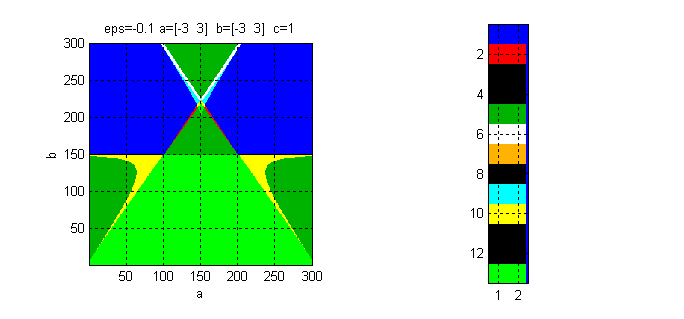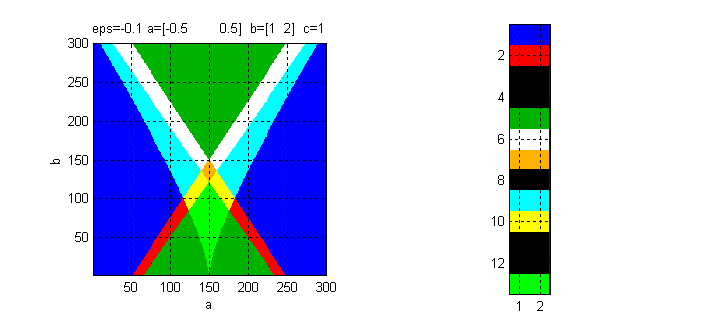Edge of chaos - example and programs implemneting some of
the concepts in the book for the FitzHugh Nagumo cell:

Run the edge_fhn file  (using Matlab or Octave)

The figure displays some "edge of chaos" sections obtained using the above program (.):
A specific "gene" (cell parameter point) can be programmed into the rd_cell.m file in  above.
Then, the simulation of the resulting RD_CNN is done using the rd_cnn.m file.
For instance rd_cnn(0,'init_18'); colormap gray, would reveal Fig.15(5b) in
reference paper:

R. Dogaru and L.O. Chua, 1998, “Edge of Chaos and Local Activity Domain of FitzHugh-Nagumo Equation”,
International Journal of Bifurcation and Chaos, Vol. 8, No. 2, 1998, pp. 211-257.